## 1. Overview

In this tutorial, we’ll discuss the Segment Tree method, which is a tree data structure. Then we’ll explore some examples.

## 2. Definition of the Segment Tree Method

The segment tree is a type of data structure from computational geometry. Bentley proposed this well-known technique in 1977. A segment tree is essentially a binary tree in whose nodes we store the information about the segments of a linear data structure such as an array.

Furthermore, it helps us solve questions on range queries along with updates. This method enables the execution of interval or range queries in logarithmic time, and we can utilize it when we must process numerous such queries.

It’s also possible to make this method a dynamic structure.

## 3. Understanding the Segment Tree Method With an Example

Segment Trees can be used to solve Range Min/Max & Sum Queries and Range Update Queries in O (log n) time. These problems can be easily solved with the Segment Tree technique. We can study this method by solving such a problem efficiently. Basically, a Segment Tree has only three operations:

• Building Tree: in this step, we create the structure of the segment tree variable and initialize it
• Updating Tree: during this step, we change the tree by updating the value in the array at a point or over an interval
• Querying Tree: we may use this operation to run a range query on the array

In a Segment Tree, the array is stored at the leaves of the tree, while the internal nodes store information about segments represented by its children. The internal nodes are formed in a bottom-up manner by merging the information from its children nodes.

Segment Trees are useful whenever we’re frequently working with ranges of numerical data. In this context, let’s look at an example to better understand the Segment Tree by describing each step. We want to construct a Segment Tree array that allows us to find the sum of the elements in an array.

### 3.1. Constructing a Segment Tree

We have an array, which has the values [,,,,,,], and we want to query the sum of a range. So every node in the segment tree will be the sum of its child nodes in this scenario.

Since it’s a binary tree, we know that the child nodes in array representation for nodeareand. For the sake of simplicity, we’ll treat the entire array, includingand, as one indexed array. As a result, each element in the Segment treewill be, whereis the node’s index, and the leaf node represents the elements in the array.

Below is the tree we have for the given array: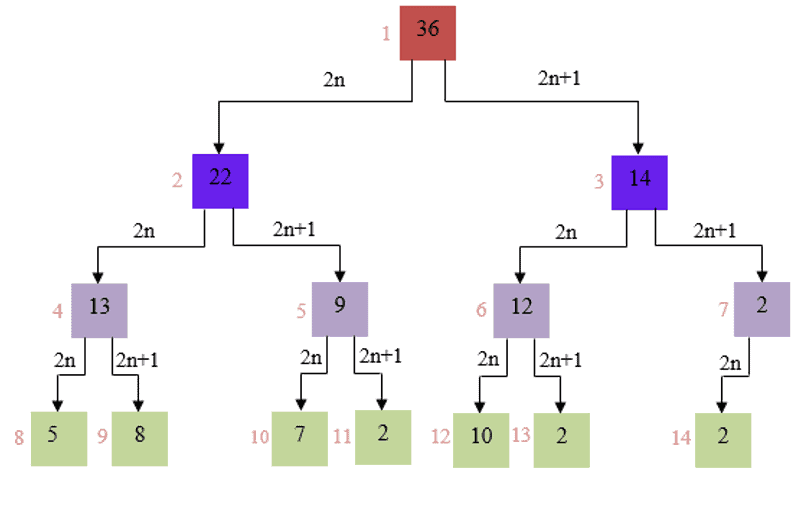The arrayis visible on the leaf of the tree: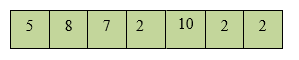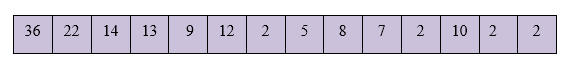The following illustration explains how a segment tree is updated.

### 3.2. Updating a Value in the Segment Tree

To update an array element, we must also update the segment tree. In fact, given that an element in the segment tree only contributes to its parent element, changing the value may be done incomplexity since we only need to traverse the height.

Therefore, to update a value, we can simply determine the diff, which is the difference between the new value of an element and the current value.

As we can see, updating the value of theth member in the array,, necessitates updating all of its parent nodes: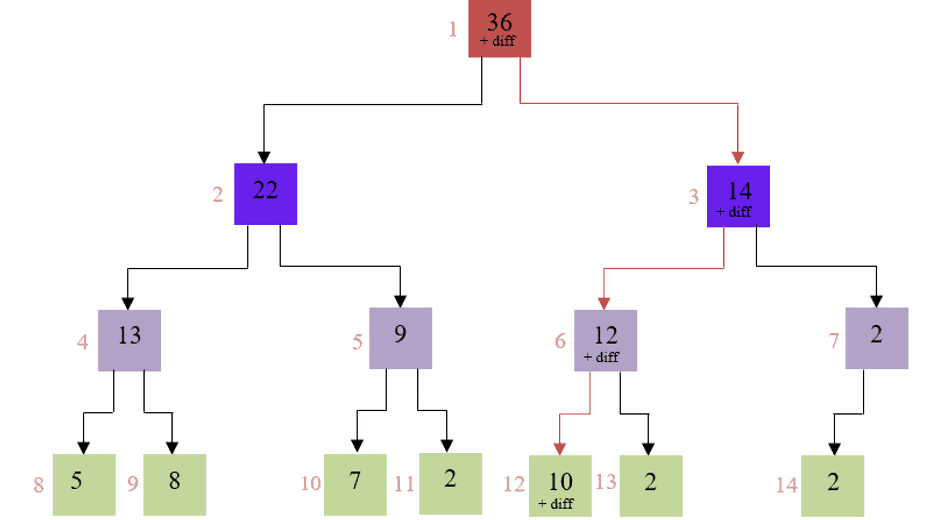The following illustration goes over how creating to run a query on a specific range.

### 3.3. Making a Range Query

Now let’s look at creating a range query using the following arrayand the Segment Treefrom the previous section.

Let’s say we want to run a sum query on a range oftoin the array. The Segment Tree can also use thecomplexity. A sum query for the range oftowill operate as shown: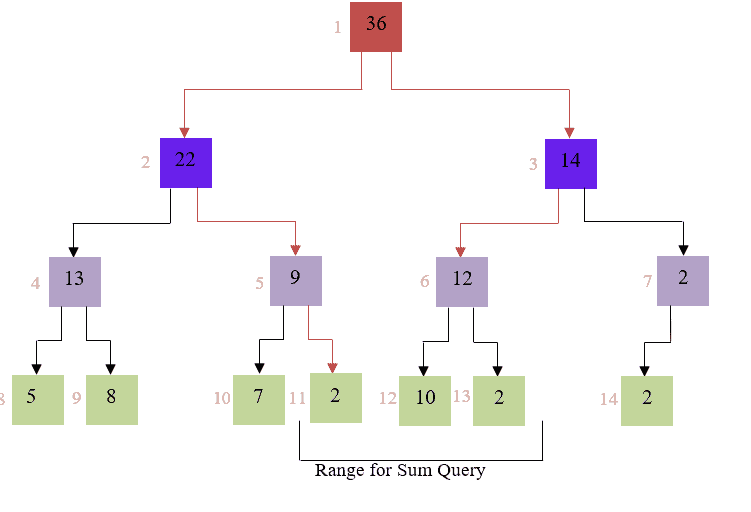We know that nodeinhas the total ofand, and thatlies in. Thus, we can write:Since the traversal is always depending on the tree’s height, it’s always.

Now let’s discuss the different cases for finding the sum of a range.

The sum of the nodes incan always be split down for a given range, such as.

As a result, while calculating the sum of a range, we can expect three scenarios:

• It’s possible that a node inisn’t providing the required sum, like,,,,. So we’ll just retrieve the value stored at that segment tree node.
• A node inmight be entirely inside the required sum, like,. Therefore, we’ll return the node’s value, which is the sum of all the elements in the range it represents.
• A node may provide a part of the required sum, like,,,. So we return the sum of the left child and the right child.

## 4. Applications of a Segment Tree

A Segment Tree is an important data structure to have in our repertoire, not only for an aspiring computer science engineer but also for any person coding as a hobby. Moreover, the Segment Tree has a vast array of possibilities and applications.

Let’s look at some applications in different areas:

• In its early days, the Segment Tree was used to efficiently list all pairs of intersecting rectangles from a list of rectangles in the plane.
• We can use this method to report the list of all rectilinear line segments in the plane which intersect a query line segment.
• We use this technique to report the perimeter of a set of rectangles in the plane.
• More recently, the segment tree has become popular for use in pattern recognition and image processing.

We can also specify other applications that are very well known:

• Finding range sum/product, range max/min, prefix sum/product, etc
• Computational geometry
• Geographic information systems
• Static and Dynamic RMQ (Range Minimum Query)
• Storing segments in an arbitrary manner

## 5. Conclusion

In this article, we learned how to create a segment tree, update a value, and make a range query that can be used to solve a range query-based problem in an efficient time. This type of data structure is advantageous and has numerous applications in different areas.